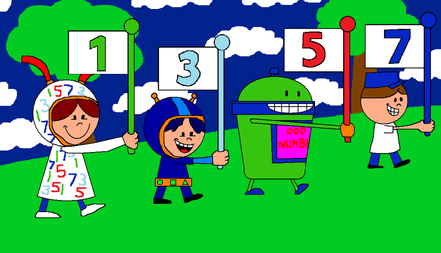Problems
501 - Odd Numbers

Odd Numbers

Time Limit: 1 sec

The Problem

Odd numbers are the numbers which cannot be divisible by 2. Example: 1, 3, 5, 7 and so on.The Input

The input file contains a series of line. Each line contains a single integer number N (0 < N <=1001). Input is terminated by EOF.

The Output

For each line of input print the all the odd numbers from 1 to N in a separate line.

Sample Input

3

7

Sample Output

1

3

1

3

5

7

Problem Setter: Samia Safa Ahmed